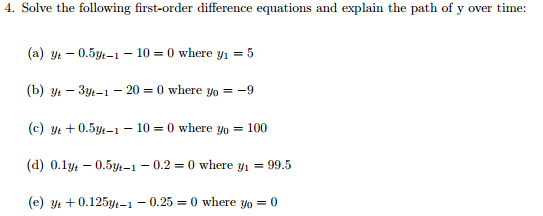### Create an Account

Home / Questions / Solve the following first-order difference equations and explain the path of y over time: ...

# Solve the following first-order difference equations and explain the path of y over time: y_t - 0.5y_t - 1 - 10 = 0 where y_1 = 5 y_t - 3y_t - 1 - 20 = 0 where y_0 = -9 y_t + 0.5y_t - 1 - 10 = 0 where

Solve the following first-order difference equations and explain the path of y over time: y_t - 0.5y_t - 1 - 10 = 0 where y_1 = 5 y_t - 3y_t - 1 - 20 = 0 where y_0 = -9 y_t + 0.5y_t - 1 - 10 = 0 where y_0 = 100 0.1y_t - 0.5y_t - ' - 0.2 = 0 where y_1 = 99.5 y_t + 0.125y_t - 1 - 0.25 = 0 where y_0 = 0Jul 08 2021 View more View LessSubscribe To Get Solution